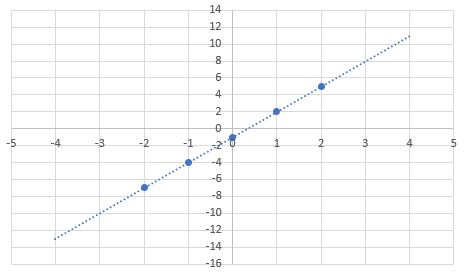# Graphing Linear Equations

To graph a linear equation, you can create a table of values (x and y) and then simply connect the points with a line. It is good practice to choose both positive and negative x values. Choose an x value and then substiute the value in the equation to solve for y. This will give you the (x,y) point for the graph.

One strategy is to start at the y-int and then use the slope to determine the next point. Let's populate a table of values for $$y = 3x - 1$$.

The y-int is at $$0,-1$$ which gives us our first points.

 X Y -2 -1 0 1 2 -1

The slope is $$+3$$ which means when x increases by 1, y increases by 3. Let's populate the table for $$x=1,2$$:

 X Y -2 -1 0 1 2 -1 2 5

Finally, to populate the other values we decrease x by 1 and decrease y by 3.

 X Y -2 -1 0 1 2 -7 -4 -1 2 5

Finally, plot the points on a coordinates grid and connect them with a line. The line extends in both directions.Graph the equation: $$y = 5(x) + 2$$

Graph the equation: $$y = -2(x) - 2$$